# Quaternion

(diff) ← Older revision | Latest revision (diff) | Newer revision → (diff)

A hypercomplex number, geometrically realizable in four-dimensional space. The system of quaternions was put forward in 1843 by W.R. Hamilton (1805–1865). Quaternions were historically the first example of a hypercomplex system, arising from attempts to find a generalization of complex numbers. Complex numbers are depicted geometrically by points in the plane and operations on them correspond to the simplest geometric transformations of the plane. It is not possible to "organize" a number system similar to the field of real or complex numbers from the points of a space of three or more dimensions. However, if one drops the requirement of commutativity of multiplication, then it is possible to construct a number system from the points of-dimensional space. (In 3, 5 or higher-dimensional space it is not even possible to do this.)

The quaternions form a-dimensional algebra over the field of real numbers with basis( "basic units" ) and the following multiplication table of the "basic units" :'

<tbody> </tbody>
 1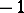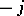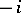Every quaternion can be written in the formor (since 1 plays the role of ordinary identity and in writing a quaternion it can be omitted) in the form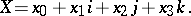One distinguishes the scalar partof the quaternion and its vector partso that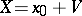. If, then the quaternionis called a vector and can be identified with an ordinary-dimensional vector, since multiplication in the algebra of quaternions of two such vectors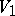and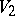is related to the scalar and vector products(cf. Inner product) and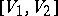(cf. Vector product) of the vectorsandin-dimensional space by the formulaThis shows the close relationship between quaternions and vector calculus. Historically, the latter arose from the theory of quaternions.

Corresponding to each quaternionis the conjugate quaternion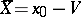, and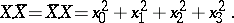This real number is called the norm of the quaternionand is denoted by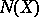. This norm satisfies the relation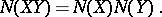Any rotation of-dimensional space about the origin can be defined by means of a quaternionwith norm 1. The rotation corresponding totakes the vector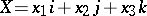to the vector.

The algebra of quaternions is the unique associative non-commutative finite-dimensional normed algebra over the field of real numbers with an identity. The algebra of quaternions is a skew-field, that is, division is defined in it, and the quaternion inverse to a quaternionis. The skew-field of quaternions is the unique finite-dimensional real associative non-commutative algebra without divisors of zero (see also Frobenius theorem; Cayley–Dickson algebra).

  L.A. Kaluzhnin, "Introduction to general algebra" , Moscow (1973) (In Russian)  I.L. Kantor, A.S. Solodovnikov, "Hyperkomplexe Zahlen" , Teubner (1978) (Translated from Russian)  A.G. Kurosh, "Higher algebra" , MIR (1972) (Translated from Russian)

Let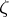be the element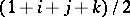in the algebra of quaternions. The Hurwitz ring of integral quaternions is the ring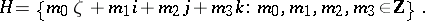The Hurwitz ring is a non-commutative ring in which an analogue of the Euclidean division property (cf. Euclidean algorithm) holds: For anywith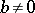there exist elements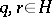such that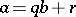with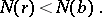(This property does not hold for the subring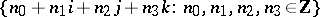.) It follows that every left ideal is left principal, and this in turn can be used to give a proof of the Lagrange four-square theorem, to the effect that every positive integer can be written as a sum of four squares of integers.

The Lagrange identity, which plays an important role in the proof of this result,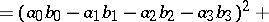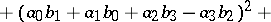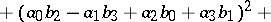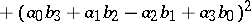for real numbers, is equivalent to the multiplicativity of the norm, where,are the quaternions,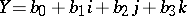.

Writingas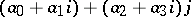and putting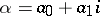,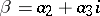, one obtains. It is easily proved that the algebra of quaternions is isomorphic to the algebra of complex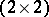-matrices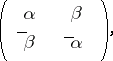with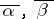the complex conjugates of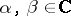.

When one wishes to retain the multiplicativity of the norm,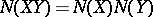, there is only one possible generalization of the quaternions (over the reals): the octaves or octonions, which have 8 instead of 4 components (Hurwitz's theorem, 1898; cf. Cayley numbers).

The centre of the skew-field of quaternions is the field of real numbers. Later the notion of hypercomplex system has been generalized in a theory of skew-fields over arbitrary fields, e.g. the theory of the Brauer group of a commutative field.

In this connection, a generalized quaternion algebra is a-dimensional algebra over a fieldgenerated by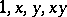with multiplication table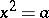,,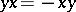, where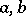are non-zero elements of. (The quaternions are the case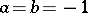, andthe field of real numbers.)

#### References

 [a1] A.A. Albert, "Structure of algebras" , Amer. Math. Soc. (1935) [a2] R. Brauer, E. Noether, "Über minimale Zerfällungskörper irreducibler Darstellungen" Sitzungsber. Akad. Berlin , 27 (1927) pp. 221–226 [a3] J.H.M. Wedderburn, "On hypercomplex numbers" Proc. London Math. Soc. Ser. 2 , 6 (1907) pp. 77–118 [a4] R. Brauer, E. Weiss, "Non-commutative rings" , Harvard Univ. Press (1950) pp. Part I [a5] H. Behnke, F. Bachmann, "Grundzüge der Mathematik" , I , Göttingen (1962) [a6] S. Maclane, G. Birkhoff, "Algebra" , Macmillan (1979) [a7] M. Crowe, "A history of vector analysis, the evolution of the idea of a vectorial system" , Univ. Notre Dame (1967) [a8] R.J. Stephenson, "Development of vector analysis from quaternions" Amer. J. Physics , 34 (1966) pp. 194–201 [a9] B.L. van der Waerden, "Hamiltons Entdeckung der Quaternionen" , Vandenhoeck & Ruprecht (1973) [a10] I.N. Herstein, "Topics in algebra" , Wiley (1975) pp. Sect. 7.4
How to Cite This Entry:
Quaternion. Encyclopedia of Mathematics. URL: http://encyclopediaofmath.org/index.php?title=Quaternion&oldid=12609
This article was adapted from an original article by N.N. Vil'yams (originator), which appeared in Encyclopedia of Mathematics - ISBN 1402006098. See original article